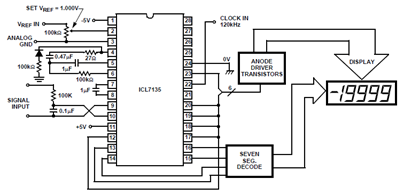# The ICL7135 Integrating A/D Converter Functional Diagram and Datasheet

The ICL7135 Integrating A/D Converter Functional Diagram and Datasheet - The schematic below shows the ICL7135 integrating analog to digital converterThe ICL7135 Integrating A/D Converter Functional Diagram and Datasheet - The schematic below shows the ICL7135 integrating analog to digital converter (ADC) functional diagram.

According to the datasheet, an integrating converter is the right choice for panel meters and digital voltmeter applications. It also can be used for reducing line frequency noise since it has very good rejection of frequencies.

In the ICL7135 datasheet, you will find sections for such the description of dual slope technique (theory and practice), simplified dual slope converter circuit diagram, analog section of dual slope converter, and more.

Get and download full pdf of this integrating A/D Converter device in the ICL7135 datasheet (source:intersil.com)

Tags: dual slope converter, ICL7135 datasheet, integrating ADC, icl7135 applications,

Related The ICL7135 Integrating A/D Converter Functional Diagram and Datasheet Posts in ADC Category
» The MSC1210 Typical External Reset Circuit Schematic
» ICL7135 Typical Application Schematic Diagram and Datasheet
» Plasma Display Circuit Diagram using ICL7135 A/D Converter
» AD8137 Analog-Digital Converter Driver Pin Configuration
» LTC1407 Analog to Digital Converter Pin Configuration and Datasheet